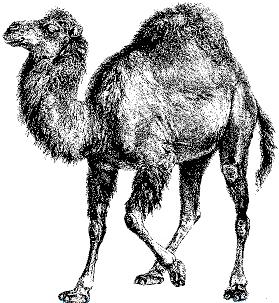# Nicholas Rinard Keene's Little Bit

I don't have a lot to say, but this is my little bit.

## Sunday, September 27, 2009

### 2D Textual Graphing With PerlHere is one way to write a simple two-dimensional graphing subroutine in perl. Here we set up an \$equation function pointer and pass it to the &printGraph subroutine, which prints a text array of dimensions \$dimension_x by \$dimension_y in the first coordinate quadrant. Each dot on the text array has size specified by \$interval_x by \$interval_y, so if you set the intervals to 1 and 1, each pixel/dot will represent a unit square.

You can play with the parameters of the script to change the size and stretch of the printed grid, and you can also modify \$equation so that it prints whatever function you want.

I was playing with this code for a while. It's easy to modify it to fill in all the area under the curve, or to print in other quadrants, or with an arbitrary range. This also isn't the only way to solve this particular problem, and I might try to show an alternate solution later.

```#!perl -w

use strict;

my \$dimension_x = 75;
my \$interval_x = 1;
my \$dimension_y = 30;
my \$interval_y = 1;

my \$equation = sub {
my \$x = shift;
return (-.01 * (\$x - 15) ** 3) + 15;
};

printGraph( \$equation );

# this subroutine takes a function reference as a parameter
sub printGraph {
my \$function = shift;

# print the Y axis and the function
for( my \$y = \$dimension_y; \$y >= 0; \$y-- ) {
printf "%4.1f", \$y * \$interval_y;
for(my \$x = 0; \$x < \$dimension_x; \$x++ ) {
# if the current pixel is close to the function, print *; else pring space
if (
( \$function->(\$x * \$interval_x) <= (\$y + .5) * \$interval_y )
and ( \$function->(\$x * \$interval_x) >= (\$y - .5) * \$interval_y)
) {
print "*";
} else {
print " ";
}
}
print "\n";
}

# print the X axis
for(my \$x = 0; \$x < \$dimension_x; \$x++ ) {
if( \$x % 8 == 0 ) {
printf "%4.1f    ", \$x * \$interval_x;
}
}
print "\n";
}```